# Flood Fill Fun

Some of the following are best viewed running live, since you then see real-time colour changes. Others create a static end-point image. I'll put up a zip file with some variations and suitable source files- or email me for specific help.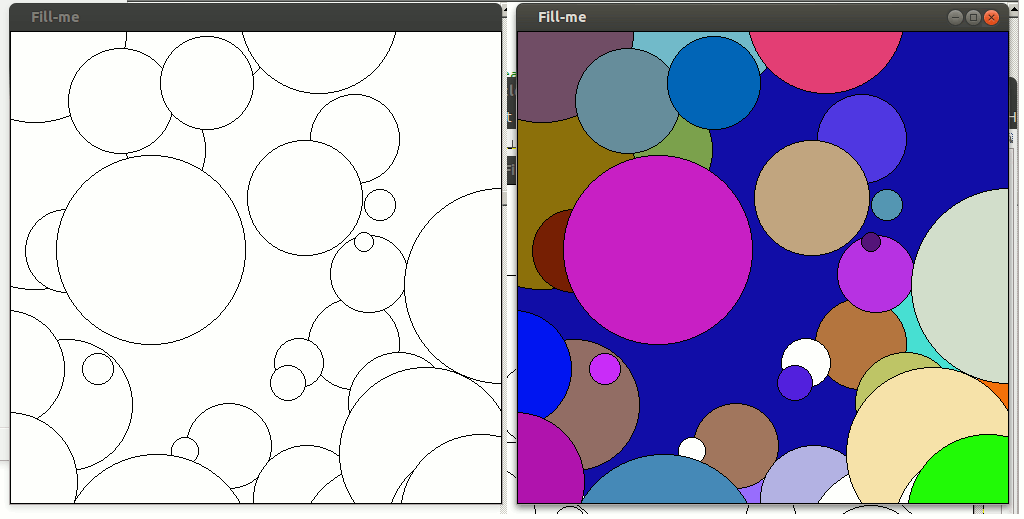I've often wanted to fill irregular-shaped graphic areas. And recently colouring-in previously prepared outline pictures has inexplicably become popular. LB allows easy calling of the flood-filling API, which can be used ( as here) to flood-fill out to a specified boundary colour OR to keep re-colouring for as long as it is in a contiguous area of stated colour. This first example could have been achieved with a 'circlefilled' command. Other shapes cannot be filled the same way...

I wrote variations of the following code which either draws a black-on-white figure, or loads a prepared BMP of the correct size. You can prepare source material from the many on-line 'colouring-in' drawings, or create them by edge-detection of suitable photos.

I choose points at random. If not black ( the chosen boundary) I fill them with a random colour out to the surrounding black boundary. Large areas are likely to get 'hit' early on and then repeatedly changed. This can be avoided by skipping if GetPixel is used to find if that area has already been coloured, but if you do that the picture will be increasingly static. Conversely, small areas may still not have been seen after many cycles. But if you use a systematic ( eg raster) scan to find areas to colour, it looks distinctly odder and less pleasing.

The next example fills a pre-supplied black outline drawing.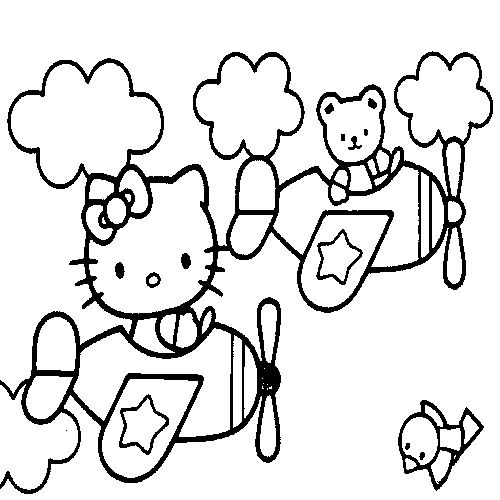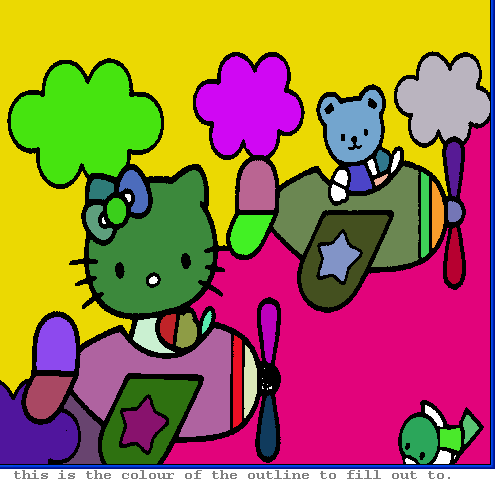And the next example colours an outlined shape at the time of its creation. Bit of a tribute to the 'Olber's Paradox' of cosmology.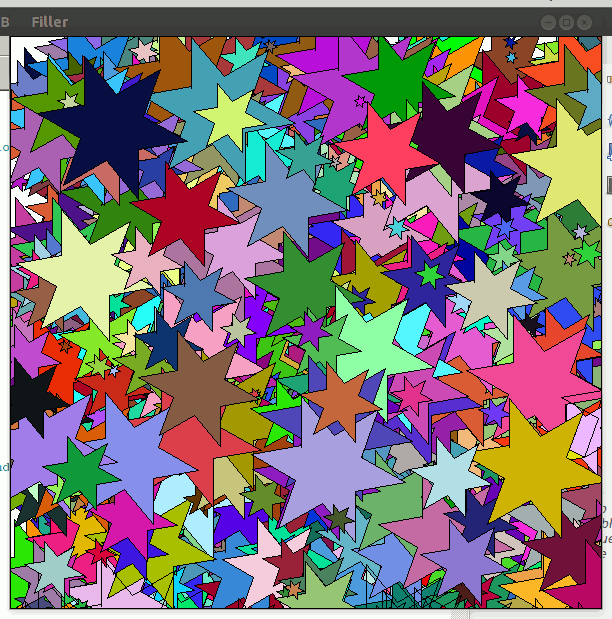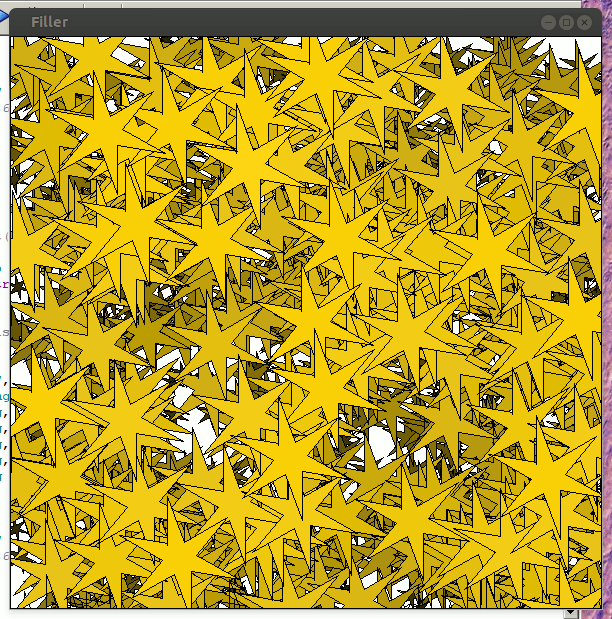### The Liberty BASIC code I used varies, but basically each time you add three bits of code. Find the graphic window handle. Call the dll. Close the dll on exit.

```nomainwin

WindowWidth  =500
WindowHeight =500

open "Fill-me" for graphics_nsb as #wg

#wg "trapclose [quit]"

hw =hwnd( #wg)	'						<<<<<<<<<<<<<<<<<<<<<<<<<<<<<<<<<<<<<<
calldll #user32, "GetDC", hw as ulong, hdc as ulong	'	<<<<<<<<<<<<<<<<<<<<<<<<<<<<<<<<<<<<<<

filedialog "Choose a 500x500 BMP", "*.bmp", fn\$

#wg "down"
#wg "drawbmp scr 0 0"
#wg "flush"

notice "Hit  to flood-fill the areas one at a time at 10/second."

for i =1 to 1000
r           =int( 256 *rnd( 1))
g           =int( 256 *rnd( 1))
b           =int( 256 *rnd( 1))
fillCol\$    =str\$( r) +" " +str\$( g); " " +str\$( b)
if fillCol\$ <>"0 0 0" then
#wg "color "; fillCol\$; " ; up ; backcolor "; fillCol\$

xVar            =int( 500 *rnd( 1))
yVar            =int( 500 *rnd( 1))
targetcolor     =0  '   this is the colour of the outline to fill out to.

calldll #gdi32, "ExtFloodFill",_
hdc                as ulong,_
xVar               as long,_
yVar               as long,_
targetcolor        as long,_
_FLOODFILLBORDER   as long,_    '   '   ie fill out 'til this colour is met... <<<<<<<<<<<<<<<<<<<<<<<<<<
result             as long

calldll #kernel32, "Sleep", 100 as long, ret as void
scan
end if
next i

#wg "flush ; getbmp scr 0 0 500 500"
bmpsave "scr", "fillDemo.bmp"

wait

[quit]
calldll #user32, "ReleaseDC", hw as ulong, hdc as ulong, ret as void   'release the DC	<<<<<<<<<<<<<<<<<<<<<
close #wg
end
```